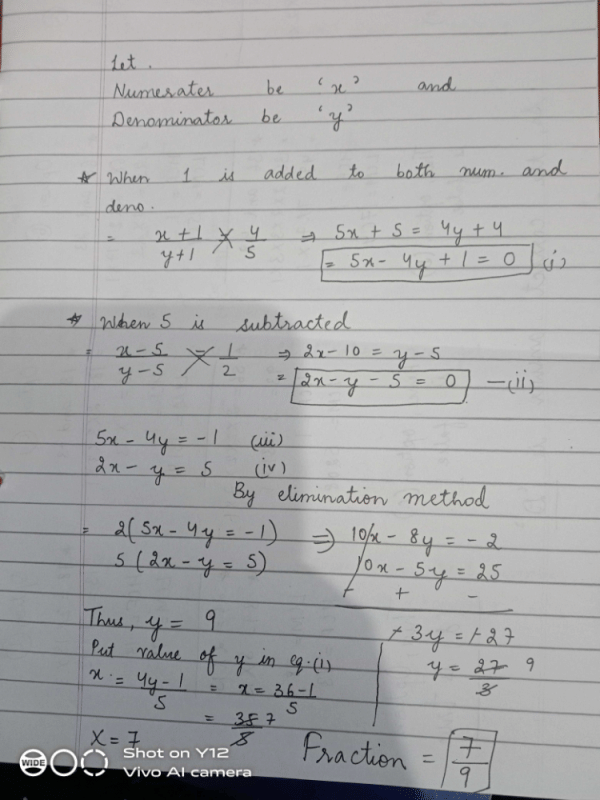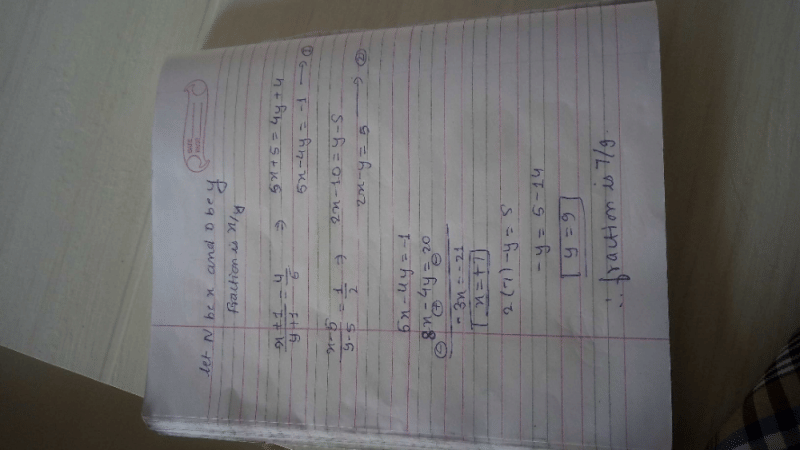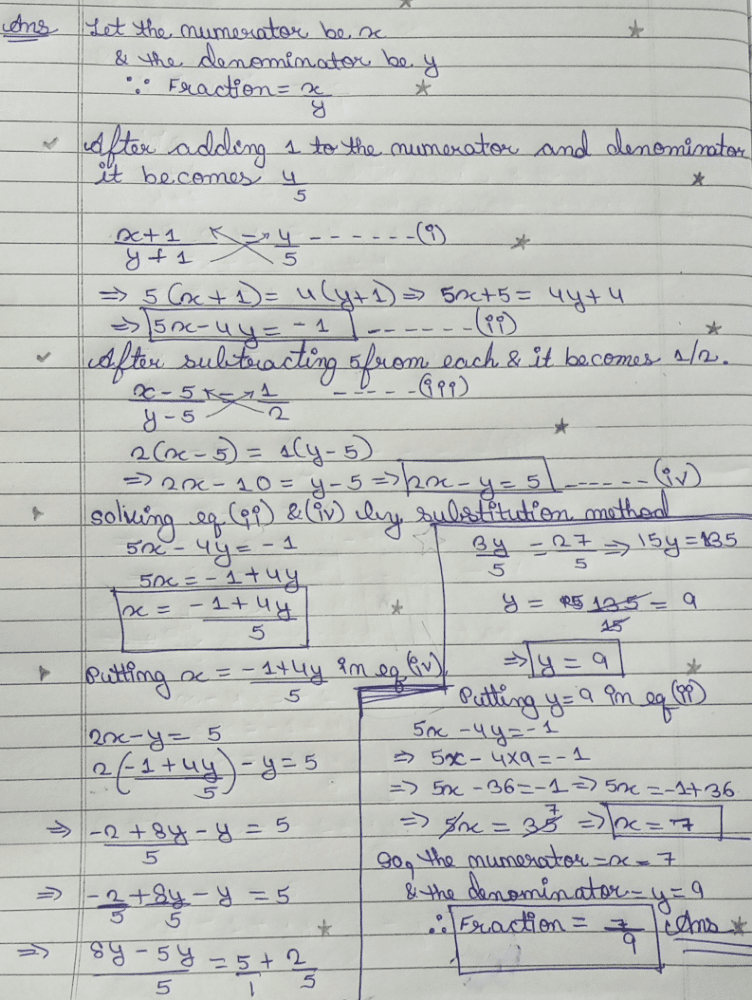A fraction becomes  4/5 when 1 is added to each of the numerator and denominator. However, if we subtract 5 from each then it becomes 1/2. The fraction is –
• a)
5/8
• b)
5/6
• c)
7/9
• d)
13/16

### Related TestRising Star Oct 12, 2020Shreya Kasaudhan Aug 11, 2020
let the fraction be x/y

x+1/y+1=4/5

5x+5=4y+4

5x-4y=-1

5 is subtracted means

x-5/y-5=1/2

2x-y=5

2 eqn multilply by 5 and 1 eqn by 2

2(5x-4y=-1)=10x-8y=-2

5(2x-y=5)=10x-5y=25

10x-5y=25

10x-8y=-2

x=7,y=9

then the fraction  is 7/9Mehak Jaju Jul 09, 2019Shweta Samaira Jan 25, 2022